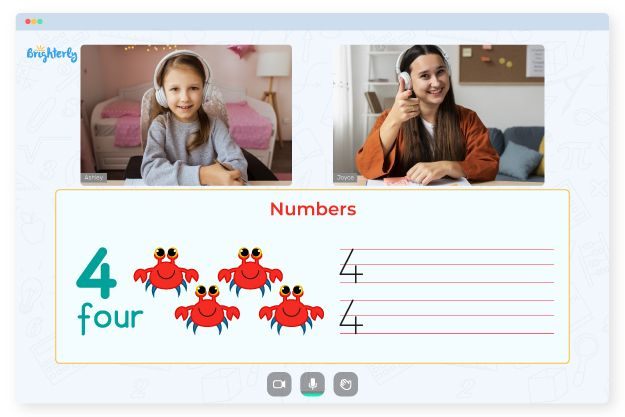# Number 2 Worksheets

Toddlers need to identify the number 2 and its place in the number line. Number 2 worksheets can help them achieve this aim thanks to their fun and interactive exercises. And since kids between the ages of 1 and 3 need constant stimulation to continue learning, number 2 worksheets are perfect tools for understanding the fundamentals of counting.

## Types of Number 2 Worksheets

Number 2 worksheets are available on Brighterly and other math platforms. You can always get a number 2 worksheet that fits the kids’ needs in your class online and use it to supplement your teaching methods. A number 2 preschool worksheet is often fun and engaging, which makes it easy to get your kids interested in the idea. Here is a list of number 2 worksheets for preschool children:

Math for Kids

Is Your Child Struggling With Math?
1:1 Online Math Tutoring### Number 2 tracing worksheet for toddlers

A number 2 tracing worksheet helps toddlers understand how the number 2 looks by tracing it on a color page. At first glance, the digit 2 seems like a complicated image to draw and may confuse kids. However, a number 2 tracing worksheet will simplify the process, making it easy for kids to understand how to write the number.

### Number 2 subtraction worksheet

Addition and subtraction are essential operations in basic math, so if you teach preschoolers to identify the number 2, those concepts will be a part of the conversation. Subtraction questions on number 2 worksheets involve two-digit numbers. These number 2 worksheets for preschool children will make it easy for kids to learn subtraction of 2s from large numbers.### Number 2

Number 2 Tracing Worksheet### Number 2 Practice

Number 2 Worksheet### Practice tracing 2’s

Number 2 Worksheet For Preschool### Number Two

Number 2 Preschool Worksheet

### Number 2 multiplication worksheet

The easiest part of the multiplication table is the number 2 table. The faster kids know how to work the number 2 in a multiplication table, the easier it will be for them to learn how to multiply other numbers. Using the number 2 multiplication worksheet, you can teach children the multiples of 2 and the way it leads to a string of even numbers.

### Numbers Worksheets

Troubles with Numbers?• Is your child finding it difficult to understand the concept of numbers?
• An online tutor could be the answer.

Does your child need additional support with numbers concepts? Start learning with an online tutor.